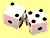Suppose you want to make card game that consists of 52 decks. Each of the cards has equal chance to be selected. Then you need to generate 52 random integers with uniform probability {1, 2, ... , 51, 52}. Another example, you want to make a program that behaves as a dice with six faces {1, 2, 3, 4, 5, 6}. Each face has equal chance to be selected. In this section, you will learn general formula to make Monte Carlo Simulation by generating N integers from lower boundary L until upper boundary B with equal chance to be selected.

If you want to generate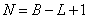random integers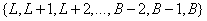that will distribute according to uniform probability, the formula is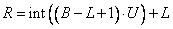Random number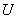that unformly distributed is generated according to previous page . To put this formula in action, I have made a worksheet in MS Excel. You may download it here . The explanation of how to use the worksheet is given below .

The expected mean is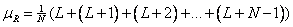Or,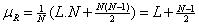The expected variance is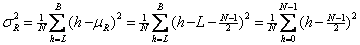or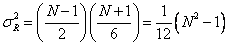### How to use the worksheet?

Worksheet companion of this tutorial can be downloaded here

The sample worksheet uses the method above to compute dice. The formulation is quite general to cover N sequence of integers with lower bound and upper bound (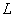and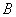) . Here is sample of results of experiments after a few hundred sampling. Notice the difference between all results and this sample.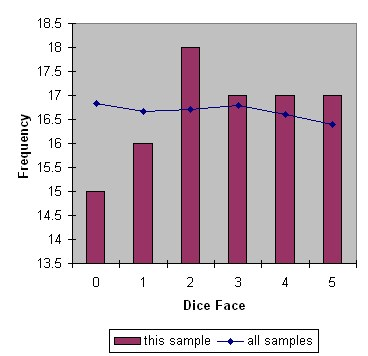Every time you press F9, it generates one sampling experiment of Monte Carlo Simulation. If you delete the word "STOP", every time you press F9, you are not only making one sample of numerical simulation; you will add the statistics of the sampling to all samples results. In this way, actually obtain full simple program of Monte Carlo Simulation.

To use the worksheet fully, delete the word "STOP" and press F9 repeatedly. If you don't delete the STOP, the simulation will still work but the result of all experiments are simply copies of the current experiment. Write any letter or number on that Blue cell to reset the counter.

The formula for mean and variance are recursive. You may see more explanation about this recursive formula in my tutorial on Recursive Statistics . If you are still confuse on how to use iteration in MS Excel, please read my tutorial on MS Excel Iteration .

Preferable reference for this tutorial is

Teknomo, Kardi. Monte Carlo Simulation Tutorial. https:\\people.revoledu.com\kardi\ tutorial\Simulation